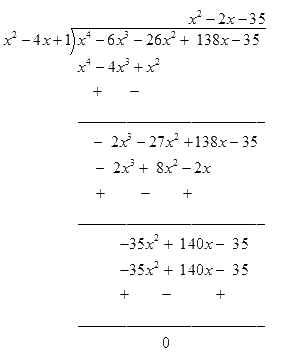# Ex.2.4 Q4 Polynomials Solution - NCERT Maths Class 10

Go back to  'Ex.2.4'

## Question

If two zeroes of the polynomial

$${x^4}-6{x^3}-26{x^2} + 138x-35$$ are $$2 \pm \sqrt 3$$

find other zeroes.

Video Solution
Polynomials
Ex 2.4 | Question 4

## Text Solution

What is known?

Two zeroes of the polynomial

$$x^{4}-6 x^{3}-26 x^{2}+138 x-35$$ are

$$2 \pm \sqrt 3 .$$

What is unknown?

Other zeroes of the given polynomial.

Reasoning:

Given polynomial and the zeroes of the polynomial are

$$x^{4}-6 x^{3}-26 x^{2}+138 x-35$$ and

$$2 \pm \sqrt 3 .$$

By using the zeroes of a polynomial, you can find out the factor of the polynomial.

Now divide the polynomial with the factor, you will get the quotient and remainder of the polynomial.

Put this value in the division algorithm and you will get the other zeroes by simplifying its factors.

Steps:

$P\left( x \right) \!= \!{x^4} \!- \! 6{x^3} \!- \!26{x^2} \!+\! 138x \! - \! 35$

Zeroes of the polynomial are $$= 2 \pm \sqrt 3 .$$

Therefore,

\begin{align}&\left( {x - 2 + \sqrt 3 } \right)\left( {x-2 - \sqrt 3 } \right) \\&= {{ }}{x^2} + 4-4x - 3\\&= {{ }}{x^2}-{{ }}4x{{ }} + {{ }}1\end{align}

is a factor of the given polynomial.

To find out the other polynomial, we have to find the quotient by dividing

$${x^4}-6{x^3}-26{x^2} + 138x-35$$ by

$${x^2}-4x + 1$$Clearly, by division algorithm,

\begin{align}&{x^4}-6{x^3}-26{x^2} + 138x-35\\&=\left( {{x^2}-4x+ 1} \right)\left( {{x^2} - 2x- 35} \right)\end{align}

It can be observed that $${x^2} - 2x - 35$$ is a factor of the given polynomial and

\begin{align}{x^2} - 2x - 35 &= {x^2} - 7x + 2x - 35\\ &= \left( {x - 7} \right)\left( {x - 5} \right)\end{align}

Therefore, the value of the polynomial is also zero when $$x-7 = 0$$ or $$x + 5 = 0$$

Or $$x=7$$ and $$x\!=\!-5$$

Hence, $$7$$ and $$\!-\!5$$ are also zeroes of this polynomial.

Learn from the best math teachers and top your exams

• Live one on one classroom and doubt clearing
• Practice worksheets in and after class for conceptual clarity
• Personalized curriculum to keep up with school